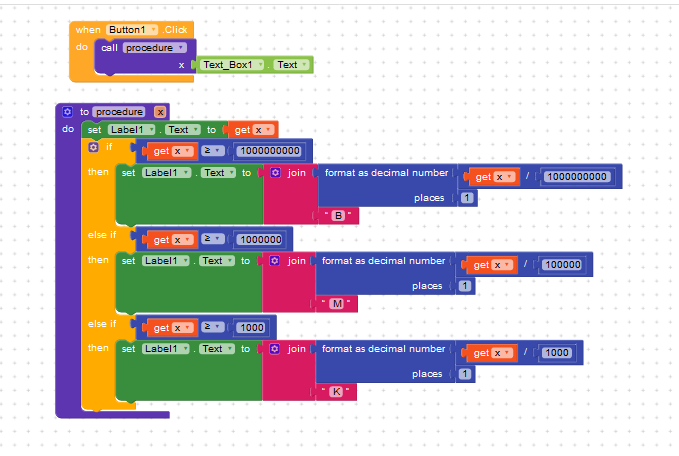# Convert number to Shortcut (1000=1K)

How to change number to Shortcut (1000=1K)
Like 1435=1.4K
Examples
1K
1.1K
547K
(K,M,B)
Use Cases: Views, Likes etc.

Is there any extension for this?
How to do this?

1 Like

i didnt seen any extension for this, maybe if exists anyone knows so they will answer,

mean while you can try to make method of you own, on github i have read that, check this link

How to implement this on Kodular?

by using if, else and else if,
and
“<” this is available in math blocks

@krishjha07 if you are free then this is a good idea to make extension.2 Likes

If you want to make extension
if it is a single number
I want 1.1K Format (with 1 decimal place)
else 765K format

convert.aia (2.9 KB)

Check This

2 Likes

We need an extension to make 3 ifs and 3 divisions? Really?2 Likes

We want logic not extension for every thing.
Extension is only for adding some extra features and libraries

2 Likes

I would suggest that you make an algorithm that relies on the log function (actually, if the value could be negative, a log of the absolue value, after ruling out the risk one would be zero).
Then, you divide by 3 to get the multiplier symbol index from a list
large_suffix = {k, M, G, T, P, E Z, Y}

(that is using the prefix defined in SI)
If you want to go on the smaller side as well, the 1/1000, 1/1000000 etc. symbols are
small_suffix = {m, μ, n, p, f, a, z, y}

@pavi2410 wondering if this can be included to Kodular Utility Blocks